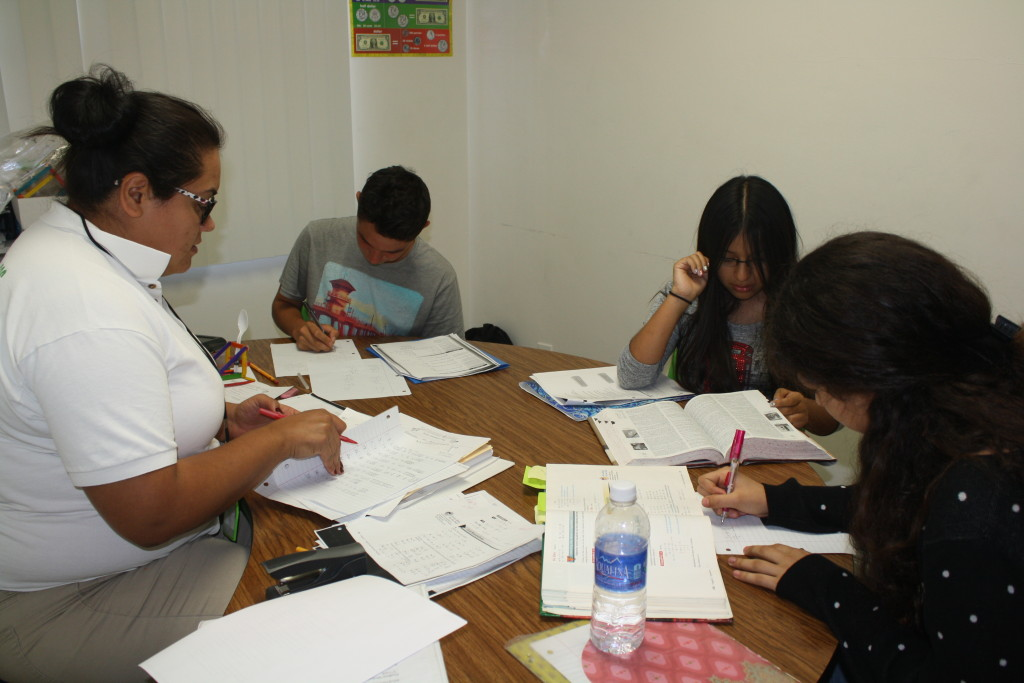# Pre-Calculus Tutoring for High School Students

##Tutoring 4 Less’ Pre-Calculus tutoring for high school students prepares them for Calculus and all other high-level mathematics by reinforcing their algebraic and problem solving skills. Students are re-exposed to the algebraic material they learned previously, in a rigorous manner, which is done with the intention of reteaching important algebra concepts that are crucial when learning calculus. Specifically, our course reintroduces students to functions, sequencing and series, counting principles, the binomial theorem, probability, data modeling and problem approaching techniques. Tutoring 4 Less also reinforces some of the most important topics of high school trigonometry including, but not limited to; graphing, curves, the polar coordinate system, complex numbers, solving problems using the Law of Sines and Cosines, vectors, conics, and Trigonometric Identities.

## Online Tutoring For Pre-Calculus

Online tutoring is here, and it’s here to stay beyond 2020. Some students learn pre-calc best through private precalculus tutoring sessions instead of a traditional classroom setting. However, private tutoring programs can be impractical for families for a number of reasons, particularly during a pandemic when in-person instruction is highly restricted in Los Angeles county. That’s why Tutoring 4 Less offers an online math tutoring experience. We do our best to match learning styles with complementary teaching styles, and our experienced tutors are well-equipped to provide all the support your student needs to improve their results. At all grade levels, from elementary to middle school to high school, academic achievement is improved by the support of Tutoring 4 Less’ highly qualified and experienced tutors.## Is Pre-Calculus Hard?

Math Analysis, more commonly known as Precalculus, is a course in high school that builds on learned content from previous mathematical courses, specifically, Algebra and Trigonometry. These math subjects help students set a strong foundation for Calculus and all other college-level math courses. It can be among the most intellectually challenging math class high school students work on. Whether it is ‘hard’ or not depends on the support a student is given, and how that support plays to the student’s strengths and learning style. Students are exposed to a number of real-world applications and are expected to master the applications and techniques for solving these multi-step problems. Graphs, diagrams, and different illustrations are used throughout this course to help students visualize some of the harder concepts that they are expected to master. A graphing utility is also introduced to help students solve the problems.

By the end of our program, students will master the algebraic techniques needed in Calculus and know all of the mathematical terminology needed in order to be successful in Calculus.  Some of the key terms students in our program will learn and understand are are:

• Asymptote: A line or curve that approaches a given curve.
• Complex Number: A number consisting of a real part and an imaginary part. A complex number is an element of the complex plane.
• Complex Plane: The set of all complex numbers. Just as all real numbers can be imagined as sitting on a line, all complex numbers can be thought of as points in a plane.
• Curve: A continuous map from a one-dimensional space to an n-dimensional space. Loosely speaking, the word “curve” is often used to mean the function graph of a two- or three-dimensional curve.
• Domain: The set of values for which a function is defined.
• Ellipse: A conic section with eccentricity less than one. It resembles a squashed circle.
• Function: A relation that uniquely associates members of one set with members of another set. The term “function” is sometimes implicitly understood to mean continuous function, linear function, or function into the complex numbers.
• Hyperbola: A conic section with eccentricity greater than one. A hyperbola consists of two separate branches.